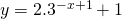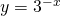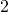# Solution assignment 08 Exponential functions and graphs

### Assignment 8

Draw the graph of the function:What is the formula of the asymptote?

### Solution

In order to draw the graph of this function we start with the graph of:When we shift this graph byto the right, multiply it byand at last shift it upwards bywe get the result.
Verify this in the figure.0